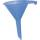# Cube root + third power - math problems

#### Number of problems found: 65

• FunnelThe funnel has the shape of an equilateral cone. Calculate the surface wetted with water if we poured into the funnel 7.1 liters of water.
• Prism XThe prism with the edges of the lengths x cm, 2x cm, and 3x cm has volume 20250 cm3. What is the area of the surface of the prism?
• BallsThree metal balls with volumes V1=71 cm3 V2=78 cm3 and V3=64 cm3 melted into one ball. Determine it's surface area.
• PowerNumber left(sqrt(14 * sqrt[ 4 ] (14)) right) 17 can be written in the form 14^x. Find the value of x.
• Plasticine ballPlasticine balls have radius r1=85 cm, r2=60 mm, r3=59 cm, r4=86 cm, r5=20 cm, r6=76 mm, r7=81 mm, r8=25 mm, r9=19 mm, r10=14 cm. For these balls

We apologize, but in this category are not a lot of examples.
Do you have an exciting math question or word problem that you can't solve? Ask a question or post a math problem, and we can try to solve it.

We will send a solution to your e-mail address. Solved examples are also published here. Please enter the e-mail correctly and check whether you don't have a full mailbox.

Cube root - math problems. Third power - math problems.